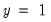predict
Prediction table for binary and ordered dependent variable models.
The prediction table displays the actual and estimated frequencies of each distinct value of the discrete dependent variable.
Syntax
eq_name.predict(n, options)
For binary models, you may optionally specify how large the estimated probability must be to be considered a success (). Specify the cutoff level as the first option in parentheses after the keyword predict.
Options

 n (default=.5) Cutoff probability for success prediction in binary models (between 0 and 1). prompt Force the dialog to appear from within a program. p Print the prediction table.
Examples
equation eq1.binary(d=l) work c edu age race
eq1.predict(0.7)
Estimates a logit and displays the expectation-prediction table using a cutoff probability of 0.7.
Cross-references
See “Binary Dependent Variable Models” for a discussion of binary models, and “Expectation-Prediction (Classification) Table” for examples of prediction tables.